# Texas Go Math Grade 6 Lesson 11.1 Answer Key Modeling Equivalent Expressions

Refer to our Texas Go Math Grade 6 Answer Key Pdf to score good marks in the exams. Test yourself by practicing the problems from Texas Go Math Grade 6 Lesson 11.1 Answer Key Modeling Equivalent Expressions.

## Texas Go Math Grade 6 Lesson 11.1 Answer Key Modeling Equivalent Expressions

Essential Question
How can you write algebraic expressions and use models to decide if expressions are equivalent?

Explore Activity
Modeling Equivalent Expressions
Equivalent expressions are expressions that have the same value.

The scale shown to the right is balanced.
A. Write an expression to represent the circles on the left side of the balance. ______
B. The value of the expression on the left side is ____.
C. Write an expression to represent the circles on the right side of the balance. ____
D. The value of the expression on the right side is ____.
E. Since the expressions have the same value, the expressions are ____.
F. What will happen if you remove a circle from the right side of the balance?
___________
G. If you add a circle to the left side of the balance, what can you do to the right side to keep the scale in balance?

Reflect

Question 1.
What If? Suppose there were 2 + 5 circles on the right side of the balance and 3 on the left side of the balance. What can you do to balance the scale? Explain how the scale models equivalent expressions.
left side = right side to make the scale balance
3 + n = 2 – 5 subtract 3 to both sides of the equation
3 + n – 3 = (2 + 5) – 3 perform the operation inside the parenthesis
n = 7 – 3 subtract the numbers
n = 4 number of circles to be added on the left side
4 circles must be added on the left side to balance the scale.

Write each phrase as an algebraic expression.

Question 2.
n times 7 ____
The algebraic expression for the phrase is in.
The expression is 7n.

Question 3.
4 minus y ____
Solution to this example is given below
The difference of 4 and y The operation is subtraction.
The algebraic expression is 4 – y (Final solution)
4 – y

Question 4.
Solution to this example is given below
The sum of 13 and x. The operation is addition. (Final solution)
The algebraic expression is 13 + x
13 + x

Write a phrase for each expression.

Question 5.
$$\frac{x}{12}$$ ______
Solution to this example is given below
$$\frac{x}{12}$$ The operation is Division.
The quotient of x and 12 (Final solution)

Question 6.
10y ____
Solution to this example is given below
10y The operation is Multiplication. (Final solution)
The product of 10 and y

Draw a bar model to represent each expression.

Question 7.
t – 2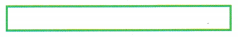Bar model of the expression: t – 2: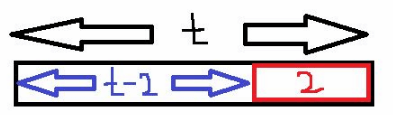Question 8.
4yBar model of the expression: 4y: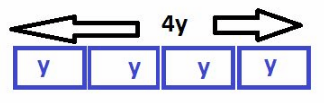Question 9.
On a math quiz, Tina scored 3 points more than Julia. Juan scored 2 points more than Julia and earned 2 points in extra credit. Write an expression and draw a bar model to represent Tina’s score and Juan’s score. Did Tina and Juan make the same grade on the quiz? Explain.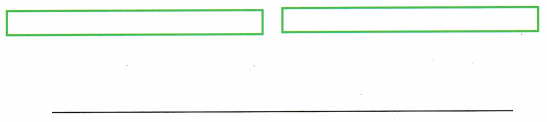Let Julia’s score be x, then Tina’s score is x + 3 and Juan’s score is x + 2 + 2 = x + 4. It can be seen that Juan’s score is not same as Tin a’s but more by 1 point.

Question 1.
Write an expression in the right side of the scale that will keep the scale balanced. (Explore Activity)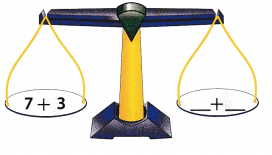The following expressions will make the scale balance:
a. 5 + 5
b 1 + 9
c. 2 + 8
d. 4 + 6

Expressions to balance the scale: 5 + 5, 1 + 9, 2 + 8, 4 + 6

Write each phrase as an algebraic expression. (Example 1)

Question 2.
3 less than y _____________________
Solution to this example is given below
The difference of y and 3. The operation is subtraction. (Final solution)
The algebraic expression is y – 3
y – 3

Question 3.
The product of 2 and p ___________
Solution to this example is given below
The product of 2 and The operation is multiplication (Final solution)
The algebraic expression is 2 × p

Write a phrase for each algebraic expression. (Example 1)

Question 4.
y + 12 ______
Solution to this example is given below
y + 12 The operation is Addition. (Final solution)
The sum of y and 12

Question 5.
$$\frac{p}{10}$$ ____
Solution to this example is given below
$$\frac{p}{10}$$ The operation is Division. (Final solution)
The quotient of p and 10

Question 6.
Draw a bar model to represent the expression m ÷ 4. (Example 2)Bar model where is the big black box and each blue box is equal to $$\frac{m}{4}$$ = m ÷ 4 = m/4At 6 p.m., the temperature in Phoenix, AZ, t, is the same as the temperature in Tucson, AZ. By 9 p.m., the temperature in Phoenix has dropped 2 degrees and in Tucson it has dropped 4 degrees. By 11 p.m., the temperature in Phoenix has dropped another 3 degrees. (Example 3)

Question 7.
Represent the temperature In each city with an algebraic expression and a bar model.Representation of the problem and algebraic expression.
Phoenix = t – 2 – 3
Tucson = t – 4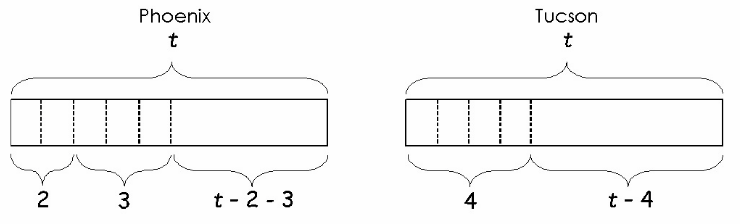The algebraic expression for Phoenix is t – 2 – 3 and Tucson is t – 4.

Question 8.
Are the expressions that represent the temperatures in the two cities equivalent? Justify your answer.
From the solution of question 6, it can be seen that the 2 temperatures are not equivalent as the temperature in Phoenix has dropped by a total of 5 degrees while that in Tucson, only dropped by 4 degrees.

Essential Question Check-In

Question 9.
How can you use expressions and models to determine if expressions are equivalent?
Algebraic expressions and models can easily determine that expressions are equivalent. Models are used to represent the word problems by graphical representation while algebraic expressions are used by translating the word problem into variables and constant

Models are graphical representation of the word problem while algebraic expression is a translation using variables and constant.

Question 10.
Write an algebraic expression with the constant 7 and the variable y.
Solution to this example is given below
The product of 7 and y. The operation is multiplication (Final solution)
The algebraic expression is 7 × y
7 × y

Question 11.
Write an algebraic expression with two variables and one constant.
The algebraic expression is m(n + 5).
Expression is m(n + 5).

Question 12.
What are the variables in the expression x + 8 – y?
Variable is a part of an algebraic expression which is represented by a single letter. Therefore, the variables ¡n the given expression are x and y.

The variables are x and y.

Question 13.
Identify the parts of the algebraic expression x + 15.
Constant(s) ________
Variable(s) _______
The parts of an algebraic expression from the given are:

• Constant: 15
• Variable: x

The constant is 15 and the variable is x.

Write each phrase as an algebraic expression.

Question 14.
n divided by 8 ______
The word divided indicates division and so the algebraic expression for the given phrase is or $$\frac{n}{8}$$ or n ÷ 8.
$$\frac{n}{8}$$ or n ÷ 8

Question 15.
p multiplied by 4 _______
Solution to this example is given below
The product of p and 4. The operation is multiplication (Final solution)
The algebraic expression is p × 4
p × 4

Question 16.
b plus 14 _______
Solution to this example is given below
The sum of b and 14 The operation is addition. (Final solution)
The algebraic expression is b + 14
b + 14

Question 17.
90 times x ______
Solution to this example is given below
The product of 90 and x. The operation is multiplication (Final solution)
The algebraic expression is 90 × x
90 × x

Question 18.
a take away 16 ______
Solution to this example is given below
The difference of a and 16 The operation is subtraction. (Final solution)
The algebraic expression is a – 16
a – 16

Question 19.
k less than 24 ______
Solution to this example is given below
k lees than 24 The operation is subtraction. (Final solution)
The algebraic expression is 24 – k
24 – k

Question 20.
3 groups of w _____
Solution to this example is given below
3 groups of w The operation is multiplication (Final solution)
The algebraic expression is 3 × w
3 × w

Question 21.
the sum of 1 and q ____
The word sum indicates addition and so the algebraic expression for the given phrase is 1 + q.
1 + q

Question 22.
the quotient of 13 and z ____
The word quotient indicates division and so the algebraic expression for the given phrase is $$\frac{13}{z}$$ or 13 ÷ z.
$$\frac{13}{z}$$ or 13 ÷ z.

Question 23.
Solution to this example is given below
The algebraic expression is c + 45
c + 45

Write a phrase in words for each algebraic expression.

Question 24.
m + 83 __________________
m + 83 can be explained by the phrase, sum of m and 83.
Sum of m and 83.

Question 25.
42s _______
42s can be explained by the phrase, Product of 42 and s.
Product of 42 and s.

Question 26.
$$\frac{9}{d}$$ _______
Solution to this example is given below
$$\frac{9}{d}$$ The operation is Division. (Final solution)
The quotient of 9 and d

Question 27.
t – 29 _____
t – 29 can be explained by the phrase, 29 less than t.
29 less than t.

Question 28.
2 + g ____
Solution to this example is given below
2 + g The operation is Addition. (Final solution)
The sum of 2 and g

Question 29.
11x ______
Solution to this example is given below
The operation is Multiplication. (Final solution)
The product of 11 and x

Question 30.
$$\frac{h}{12}$$ _______
Solution to this example is given below
$$\frac{h}{12}$$ The operation is Division. (Final solution)
The quotient of h and 12

Question 31.
5 – k ____
5 – k can be explained by the phrase, k less than 5.
k less than 5.

Sarah and Noah work at Read On Bookstore and get paid the same hourly wage. The table shows their work schedule for last week.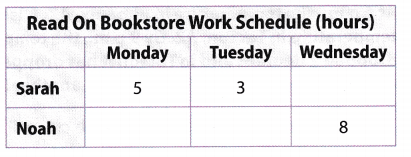Question 32.
Write an expression that represents Sarah’s total pay last week. Represent her hourly wage with w.
She worked for 3 + 5 = 8 hours and her pay is $w per hour so lier total pay for 8 hours will be 8 × w = 8w. Her total pay for 8 hours will be$8w.

Question 33.
Write an expression that represents Noah’s total pay last week. Represent his hourly wage with w.
He worked for 8 hours and his pay is $w per hour so his total pay for 8 hours will be 8 × w = 8w. His total pay for 8 hours will be$8w.

Question 34.
Are the expressions equivalent? Did Sarah and Noah earn the same amount last week? Use models to justify your answer.
Yes, both the expressions are equivalent. This is because both of them worked for 8 hours each so earned a total of $8w each. Question 35. Critique Reasoning Lisa concluded that 3 • 2 and 32 are equivalent expressions. Is Lisa correct? Explain. Answer: Lisa is incorrect because 3 . 2 ≠ 32 3 . 2 = 6 2 is a factor 32 = 3 × 3 2 is an exponent which multiplies the base by itself twice = 9 Lisa is incorrect because the value of the expressions will not be equal Question 36. Multiple Representations How could you represent the expressions x – 5 and x – 3 – 3 on a scale like the one you used in the Explore Activity? Would the scale balance? Answer: x – 5 and x – 3 – 3 By looking at the algebraic expressions and evaluating them, the scale is not balanced because the constant term on the right side of the expression is -6. while the constant term on the left side is -5. In order to make the expressions balanced, the value of x for both sides will be different. The value of x on the left side must be 10 while the value of x on the right, side must be 11 to make the scale balance.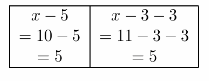The value of x for both sides will be different to make the scale balanced. Question 37. Multistep Will, Hector, and Lydia volunteered at the animal shelter in March and April. The table shows the number of hours Will and Hector volunteered in March. Let x represent the number of hours Lydia volunteered in March.a. Will’s volunteer hours in April were equal to his March volunteer hours plus Lydia’s March volunteer hours. Write an expression to represent Will’s volunteer hours in April. Answer: Will’s volunteer hours in April is equal to the 3 + x hours, as he volunteered for the sum of the number of hours he did in March and the number of hours that Lydia volunteered for b. Hector’s volunteer hours in April were equal to 2 hours less than his March volunteer hours plus Lydia’s March volunteer hours. Write an expression to represent Hector’s volunteer hours in April. Answer: Hector’s volunteer hours in April is equal to the (5 – 2) + x = 3 + x hours, as he volunteered for the sum of the number of hours he did in March minus 2 and the number of hours that Lydia volunteered for. c. Did Will and Hector volunteer the same number of hours in April? Explain. Answer: Yes, in April both of them volunteered for the same number of hours as proved by their respective expressions. Question 38. The town of Rayburn received 6 more inches of snow than the town of Greenville. Let g represent the amount of snow in Greenville. Write an algebraic expression to represent the amount of snow in Rayburn. Answer: The town of Rayburn received 6 more inches of snow than the town of Greenville. Let g represent the amount of snow in Greenville, then the town of Rayburn received 6 + g inches of snow. The town of Rayburn received 6 + g inches of snow Question 39. Abby baked 48 cookies and divided them evenly into bags. Let h represent the number of bags. Write an algebraic expression to represent the number of cookies in each bag. Answer: The number of cookies in each bag is represented by $$\frac{48}{b}$$ The algebraic expression is $$\frac{48}{b}$$. Question 40. Eli is driving at a speed of 55 miles per hour. Let h represent the number of hours that Eli drives at this speed. Write an algebraic expression to represent the number of miles that Eli travels during this time. Answer: The algebraic expression that would represent the number of hours that Eli drives is 55h where h stands for the number of hours. Expression is 55h. Texas Go Math Grade 6 Lesson 11.1 H.O.T. Focus On Higher Order Thinking Answer Key Question 41. Represent Real-World Problems If the number of shoes in a closet is s, then how many pairs of shoes are in the closet? Explain. Answer: The algebraic expression that would represent the number of pairs of shoes in the closet is $$\frac{s}{2}$$ where s stands for the number of shoes Since a pair of shoes consists of 2 shoes, that is why the total number of shoes in the closet will be divided by 2 to get the number of pairs of shoes. Expression is $$\frac{s}{2}$$ Question 42. Communicate Mathematical Ideas Is 12x an algebraic expression? Explain why or why not. Answer: The given expression is an algebraic expression because it consists of a variable and a coefficient. The coefficient is 12 and the variable is x. It represents the mathematical phrase, the product of 12 and x Yes, it represents the product of 12 and x Question 43. Problem Solving Write an expression that has three terms, two different variables, and one constant. Answer: An expression that has three terms, two different variables, and one constant is 5x + 3y + 2 a real world problem to represent this expression is a total food bill of x sandwiches each costing$5 and y drinks each costing $3 and a tip of$2.

Question 44.
Represent Real-World Problems Describe a situation that can be modeled by the expression x – 8.